# 4. Determining Object Masks

## 4. Determining Object Masks

The principle of our segmentation algorithm is to compute the difference of the current frame to a scene background image which does not contain any foreground objects. The background image is automatically constructed from the sequence such that the background adapts itself to changes or varying illumination. Even if the background is never visible without any foreground objects, the algorithm is capable to artificially recreate it.

Since the difference between input image and background contains much error due to camera noise or small parts of the object having the same color as the background, a regularization of the object shape is applied to the difference frame.

### 4.1 Background Reconstruction

The motion estimation step provides the motion model θj,j+1 between consecutive frames j and j + 1. By considering the transitive closure as the concatenation of motion transformations, we can define all θj,k between arbitrary frames j, k. If we fix the first frame as the reference coordinate system for the background reconstruction, we can add frame j to the background by applying the transformation θ1,j. To prevent the drift from slight errors in the motion estimation step, the direct estimation step is not applied to successive frames but to the input frame with respect to the current background mosaic. Figure 23.10 shows how input frames are assembled into a combined mosaic.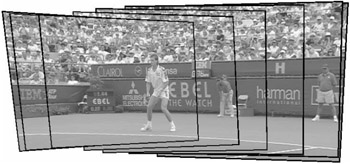Figure 23.10: Reconstruction of background based on compensation of camera motion between video frames. The original video frames are indicated with borders.

In general, the input video will contain foreground objects in most of the frames. However, it is important that the reconstructed background does not contain these objects. As it is not apriori clear which parts are foreground and which are background, we define everything as background that is stable for at least b frames. The reconstruction algorithm stores the last 2b background mosaics obtained so far. The reconstructed background image is then determined by applying a temporal median filter [12,21] over these pictures (cf. Figure 23.11). Clearly, if at least b pictures have nearly the same color at a pixel, this value will be set in the background reconstruction.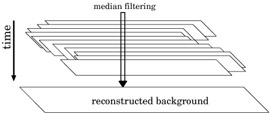Figure 23.11: Aligned input frames are stacked and a pixel-wise median filter is applied in the temporal direction to remove foreground objects.

This approach works well if the objects are moving in the scene. If they stay too long at the same position, they will eventually become background. A sample reconstructed background from the "stefan" sequence can be seen in Figure 23.20.

### 4.2 Change Detection Masks

The principle of our segmentation algorithm is to calculate the change detection mask (CDM) between the background image and the input frames. In the area where the foreground object is located, the difference between background and input frame will be high. Note that the approach of taking the difference to a reconstructed background has several advantages over taking differences between successive frames:

1. The segmentation boundaries are more exact. If differences are computed between successive frames, not only the new position of an object will have large differences, but also the uncovered background areas. This results in annoying artifacts because fast moving objects are visible twice.

2. Objects that do not move for some time or that are only moving slowly can not be segmented. Moreover, a slowly moving region with almost uniform color would only show differences at the edges in successive frames.

3. The reconstructed background can be used for object-based video coding algorithms like MPEG-4 where the background can be transmitted independently (as a so called "background-sprite"), which reduces the required bit-rate as only the foreground objects have to be transmitted.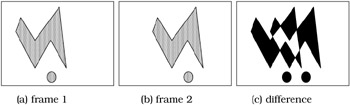Figure 23.12: Computing the difference between successive frames results in unwanted artifacts. The first two pictures show two input frames with foreground objects. The right picture shows the difference. Two kinds of artifacts can be observed. First, the circle appears twice since the algorithm cannot distinguish between appearing and disappearing. Second, part of the inner area of the polygon is not filled because the pixels in this area do not change their brightness.

### 4.3 Improved Change Detection Based on the SSD-3 Measure

With standard change detection based on squared or absolute differences, a typical artifact can be observed. If the images contain sharp edges or fine texture, these structures usually can not be cancelled completely because of improper filtering and aliasing in the image acquisition process. Hence, fine texture and sharp edges are often accidentally detected as moving objects.

One technique to reduce this effect is to use the sum of spatial distances (SSD-3) measure  to compute the difference frame. The principle of this measure is to calculate the distance that a pixel has to be moved to reach a pixel of similar brightness in the reference frame. In the one dimensional case, it is defined as

 (23.33)with

 (23.34)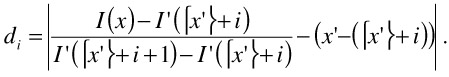For the two-dimensional case, this measure is computed independently for the horizontal and vertical direction and the minimum is taken. For an in-depth explanation of this measure, see . The difference frames obtained with this measure compared to standard squared error is depicted in Fig. 23.13.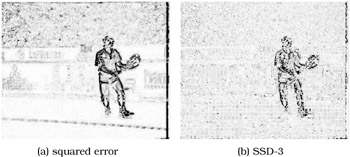Figure 23.13: Difference frames using squared error and SSD-3. Note that SSD-3 shows considerably less errors at edges caused by aliasing in the sub-sampling process.

### 4.4 Shape Regularization Using Markov Random Fields

If the generation of a binary object mask from the difference frame is done pixel by pixel with a fixed threshold, we have to face a lot of wrongly classified pixels (cf. Figure 23.15a). Since most real objects have smooth boundaries, we improve the segmentation by a shape regularization, which is done using a Markov random field (MRF) model.

The formal definition of our segmentation problem is that we want to assign a label out of the label set L={ background, foreground } to each pixel position p. For each pixel position there is a random variable Fp with values fp L. The probability that pixel p is assigned label fp is denoted as P(Fp = fp). A random field is Markovian if the probability of a label assignment for a pixel is only dependent on the neighborhood of this pixel:

 (23.35)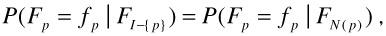where FI-{p} denotes the label configuration of the whole image except pixel p and FN(p) the configuration in a neighborhood of pixel p. We define the neighborhood of p as the 8-neighborhood, while differentiating between straight and diagonal neighbors (see Figure 23.14).Figure 23.14: Definition of pixel neighborhood. Picture (a) shows the two classes of pixel neighbors; straight (1) and diagonal (2). These two classes are used to define the second order cliques. Straight cliques (b) and diagonal cliques (c).

Since the probabilities P(Fp = fp | FN(p))are usually hard to define, Markov random fields are often modelled as Gibbs random fields (GRF). It can be shown that both descriptions are equivalent . A GRF is defined through the total label configuration probability P(f) as

 (23.36)where Z is a normalization constant to ensure that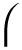f P(f) = 1. In the following, we will always set the temperature parameter T = 1. U(f) is the energy function, which is defined as

 (23.37)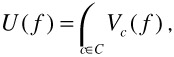in which the sum is over all cliques in the image and Vc(f) is a clique potential. Higher clique potentials result in lower probabilities for this clique configuration. Cliques are subsets of related pixel positions in the image. In our application, we are using an Auto-Logistic model, which only uses cliques of single order (the pixels themselves) and of second order (cf. Figure 23.14). Thus, the energy function can be written as

 (23.38)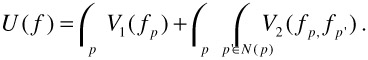First order clique potentials V1(p) are set according to the difference frame information, i.e., how probable a pixel p belongs to foreground objects given its difference frame value d(p).

 (23.39)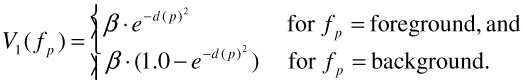The second order clique potentials are set such that smooth regions are preferred, i.e., cliques that contain different labels are assigned more energy. More specifically, we use

 (23.40)The parameter μ is set differently for the two types of cliques with lower values for diagonal cliques as the corresponding pixels are farther away. The label configuration that maximizes the total field probability (Eq.36) is obtained through an iterative Gibbs sampling algorithm . Figure 23.15b shows the segmentation mask obtained with our MRF model compared to a pixel based classification. Applying MRF-based classification to each difference frame yields binary object masks for the entire video.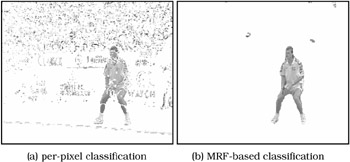Figure 23.15: Segmentation results for per-pixel decision between foreground and background object and MRF based segmentation.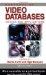Handbook of Video Databases: Design and Applications (Internet and Communications)
ISBN: 084937006X
EAN: 2147483647
Year: 2003
Pages: 393

flylib.com © 2008-2017.
If you may any questions please contact us: flylib@qtcs.net